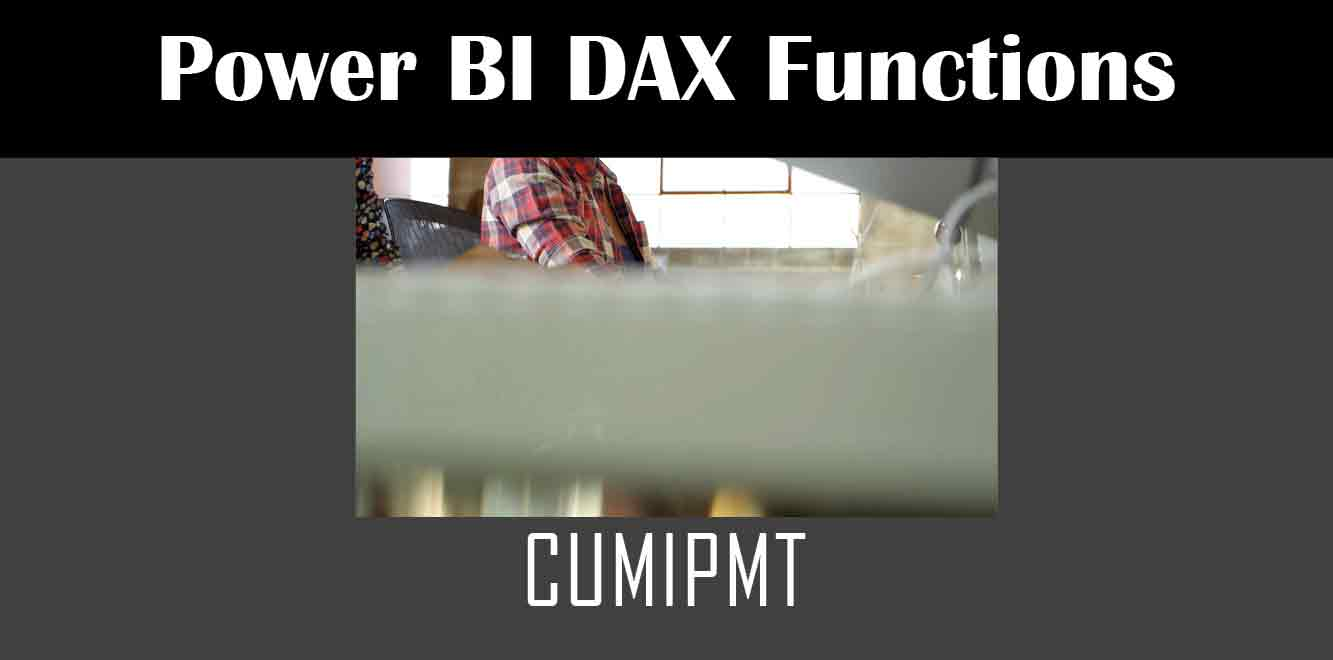# CUMIPMT

## Y## Syntax of CUMIPMT Function

The syntax for CUMIPMT function in Power BI is as follows:

CUMIPMT(rate, nper, pv, start_period, end_period, type)

``` Where: - `rate`: The interest rate per period. - `nper`: The total number of payment periods in the loan. - `pv`: The present value of the loan. - `start_period`: The period number in which the cumulative interest calculation should begin. - `end_period`: The period number in which the cumulative interest calculation should end. - `type`: The type of payment at the end of the period. 0 for end of period payments and 1 for beginning of period payments. How to Use CUMIPMT Function To use the CUMIPMT function in Power BI, follow the steps below: 1. Open Power BI Desktop and create a new report. 2. Click on the 'New Measure' option from the 'Modeling' tab in the ribbon. 3. Enter a name for the measure in the formula bar and then type the following formula: ```

=CUMIPMT([rate], [nper], [pv], [start_period], [end_period], [type])

``` 4. Replace the values in the square brackets with the appropriate values based on your loan details. 5. Press the 'Enter' key to apply the formula. Examples of using CUMIPMT Function Here are some examples that demonstrate how to use the CUMIPMT function in Power BI: Example 1 Suppose you take out a loan of \$100,000 for a period of 10 years with a 5% interest rate. You want to calculate the total interest paid for the first 5 years. The formula for this calculation is: ```

=CUMIPMT(5%/12, 10*12, 100000, 1, 5*12, 0)

``` Here, the interest rate is divided by 12 to get the monthly rate, and the total number of payment periods is calculated by multiplying the number of years by 12. The present value of the loan is \$100,000, and the calculation of the cumulative interest starts from the first month and ends at the end of the fifth year. The type of payment is end-of-period payments. The result of this formula is the total interest paid for the first 5 years, which is \$27,295.23. Example 2 Suppose you take out a loan of \$50,000 for a period of 5 years with a 7% interest rate. You want to calculate the total interest paid for the entire period. The formula for this calculation is: ```

=CUMIPMT(7%/12, 5*12, 50000, 1, 5*12, 0)

``` Here, the interest rate is divided by 12 to get the monthly rate, and the total number of payment periods is calculated by multiplying the number of years by 12. The present value of the loan is \$50,000, and the calculation of the cumulative interest starts from the first month and ends at the end of the fifth year. The type of payment is end-of-period payments. The result of this formula is the total interest paid for the entire period, which is \$17,598.23. The CUMIPMT function in Power BI is a powerful tool for calculating the cumulative interest paid on a loan. By following the steps outlined in this article, you can use this function to make informed decisions about your loan payments and better manage your finances. Power BI DAX Training Courses by G Com Solutions (0800 998 9248)Power BI DAX Intensive Training Course £1,050.00 – £26,550.00 Select optionsContinue Loading DonePower BI DAX Introduction £395.00 – £9,750.00 Select optionsContinue Loading DonePower BI DAX Intermediate £395.00 – £9,750.00 Select optionsContinue Loading DonePower BI DAX Advanced £395.00 – £9,750.00 Select optionsContinue Loading Done Upcoming Courses Contact Us Subject Your Name (required) Company/Organisation Email (required) Telephone Training Course(s) Power BI Intensive TrainingPower BI introduction Power BI IntermediatePower BI AdvancedDAXPower Query MPower BI CertificationPower BI AdministrationPower PlatformPower AutomatePower AppsOTHER Your Message Upload Example Document(s) (Zip multiple files) ```
``` ```
``` ```
``` ```
``` ```
``` ```